Question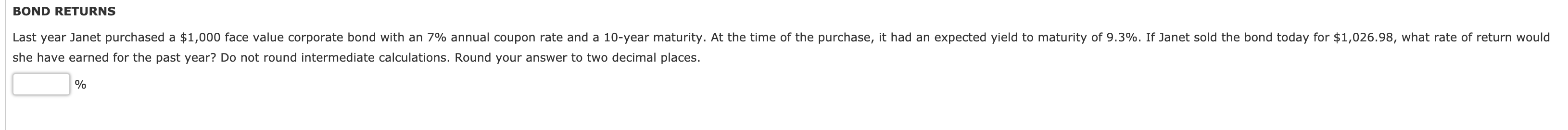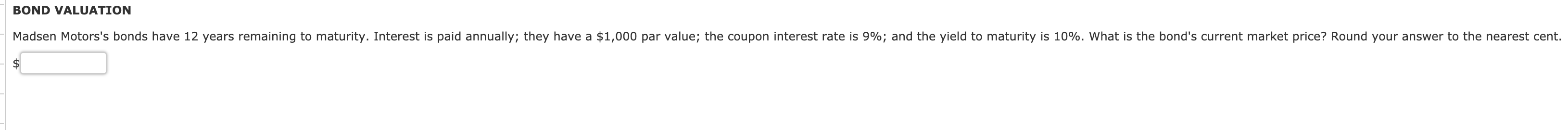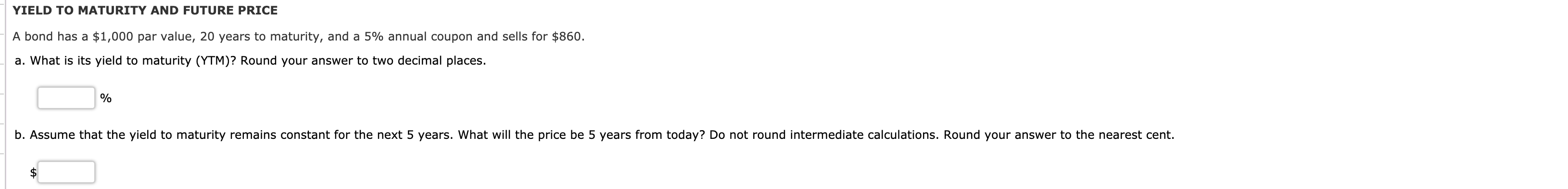Answer to Question 1:

Calculation of purchase price:

Face value = \$1,000

Annual coupon rate = 7%
Annual coupon = 7% * \$1,000
Annual coupon = \$70

Annual YTM = 9.30%
Time to maturity = 10 years

Purchase price = \$70 * PVIFA(9.30%, 10) + \$1,000 * PVIF(9.30%, 10)
Purchase price = \$70 * (1 - (1/1.093)^10) / 0.093 + \$1,000 / 1.093^10
Purchase price = \$854.32

Calculation of rate of return earned:

Purchase price = \$854.32
Coupon received = \$70
Selling price = \$1,026.98

Rate of return = (Selling price + Coupon received - Purchase price) / Purchase price
Rate of return = (\$1,026.98 + \$70.00 - \$854.32) / \$854.32
Rate of return = \$242.66 / \$854.32
Rate of return = 0.2840 or 28.40%

#### Earn Coins

Coins can be redeemed for fabulous gifts.

Similar Homework Help Questions
• ### BOND RETURNS Last year Janet purchased a \$1,000 face value corporate bond with an 7% annual...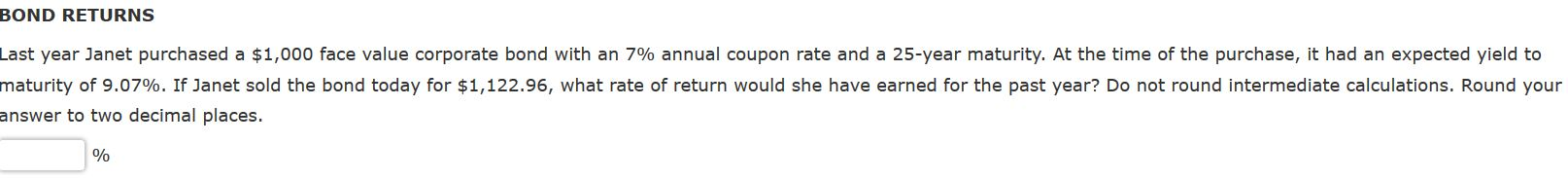BOND RETURNS Last year Janet purchased a \$1,000 face value corporate bond with an 7% annual coupon rate and a 25-year maturity. At the time of the purchase, it had an expected yield to maturity of 9.07%. If Janet sold the bond today for \$1,122.96, what rate of return would she have earned for the past year? Do not round intermediate calculations. Round your answer to two decimal places. 이

• ### Last year Janet purchased a \$1,000 face value corporate bond with an 7% annual coupon rate...

Last year Janet purchased a \$1,000 face value corporate bond with an 7% annual coupon rate and a 20-year maturity. At the time of the purchase, it had an expected yield to maturity of 8.86%. If Janet sold the bond today for \$1,145.38, what rate of return would she have earned for the past year? Do not round intermediate calculations. Round your answer to two decimal places.

• ### Last year Janet purchased a \$1,000 face value corporate bond with an 7% annual coupon rate...

Last year Janet purchased a \$1,000 face value corporate bond with an 7% annual coupon rate and a 20-year maturity. At the time of the purchase, it had an expected yield to maturity of 8.86%. If Janet sold the bond today for \$1,145.38, what rate of return would she have earned for the past year? Do not round intermediate calculations. Round your answer to two decimal places.

• ### Last year Janet purchased a \$1,000 face value corporate bond with an 9% annual coupon rate...

Last year Janet purchased a \$1,000 face value corporate bond with an 9% annual coupon rate and a 25-year maturity. At the time of the purchase, it had an expected yield to maturity of 13.45%. If Janet sold the bond today for \$1,108.92, what rate of return would she have earned for the past year? Do not round intermediate calculations. Round your answer to two decimal places.

• ### eBook Problem Walk-Through Last year Janet purchased a \$1,000 face value corporate bond with a 10%...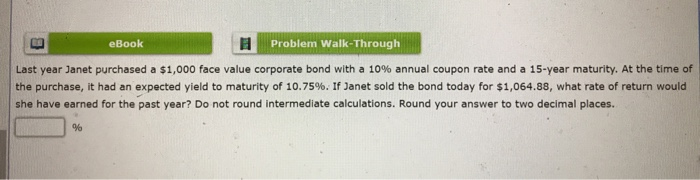eBook Problem Walk-Through Last year Janet purchased a \$1,000 face value corporate bond with a 10% annual coupon rate and a 15-year maturity. At the time of the purchase, it had an expected yield to maturity of 10.75%. If Janet sold the bond today for \$1,064.88, what rate of return would she have earned for the past year? Do not round intermediate calculations. Round your answer to two decimal places. %

• ### Bond returns Last year, Joan purchased a \$1,000 face value corporate bond with an 12% annual...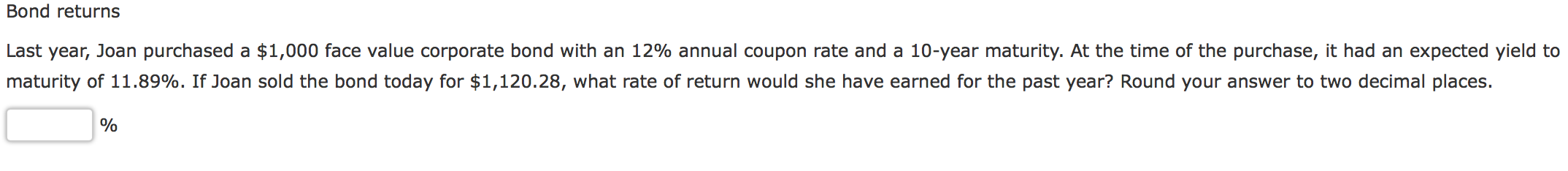Bond returns Last year, Joan purchased a \$1,000 face value corporate bond with an 12% annual coupon rate and a 10-year maturity. At the time of the purchase, it had an expected yield to maturity of 11.89%. If Joan sold the bond today for \$1,120.28, what rate of return would she have earned for the past year? Round your answer to two decimal places. 이이

• ### YIELD TO MATURITY AND FUTURE PRICE A bond has a \$1,000 par value, 20 years to...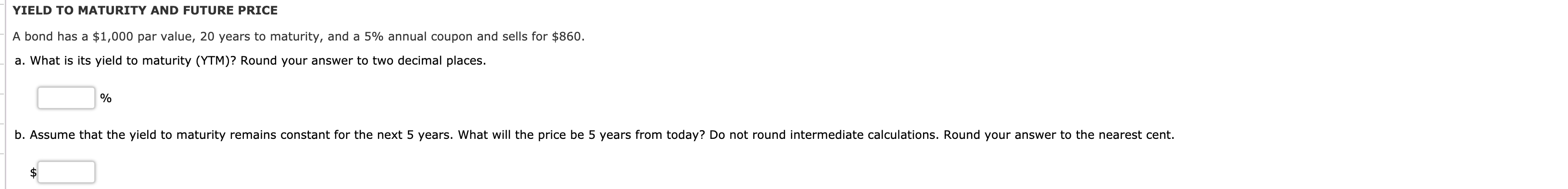YIELD TO MATURITY AND FUTURE PRICE A bond has a \$1,000 par value, 20 years to maturity, and a 5% annual coupon and sells for \$860. a. What is its yield to maturity (YTM)? Round your answer to two decimal places. b. Assume that the yield to maturity remains constant for the next 5 years. What will the price be 5 years from today? Do not round intermediate calculations. Round your answer to the nearest cent.

• ### A bond has a \$1,000 par value, 20 years to maturity, and an 8% annual coupon...

A bond has a \$1,000 par value, 20 years to maturity, and an 8% annual coupon and sells for \$1,110. What is its yield to maturity (YTM)? Round your answer to two decimal places.     % Assume that the yield to maturity remains constant for the next 5 years. What will the price be 5 years from today? Do not round intermediate calculations. Round your answer to the nearest cent. \$

• ### A bond has a \$1,000 par value, 20 years to maturity, and an 8% annual coupon...

A bond has a \$1,000 par value, 20 years to maturity, and an 8% annual coupon and sells for \$1,110. What is its yield to maturity (YTM)? Round your answer to two decimal places.     % Assume that the yield to maturity remains constant for the next 5 years. What will the price be 5 years from today? Do not round intermediate calculations. Round your answer to the nearest cent. \$

• ### A bond has a \$1,000 par value, 12 years to maturity, and a 9% annual coupon...

A bond has a \$1,000 par value, 12 years to maturity, and a 9% annual coupon and sells for \$1,110. What is its yield to maturity (YTM)? Round your answer to two d Assume that the yield to maturity remains constant for the next 3 years. What will the price be 3 years from today? Do not round intermediate calculations. Round your answer to the nearest cent.